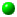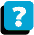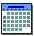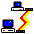Module 5.3 Notes "Multiple Sample Tests with Categorical Data"

 Index to Module 5 Notes5.1: Simple, Joint, Marginal and Conditional Probability Distributions5.2: Confidence Interval and Hypothesis Testing for a Proportion5.3: Multiple Sample Tests with Categorical Data

In Module Notes 5.2 we presented material for estimating and testing a population proportion from a single sample. This set of notes extends the methodology to the case where we want to estimate and test for the difference between two proportions, then test for the difference between multiple proportions. We conclude with a test for the relationship between two categorical variables.

Tests for the Difference in Two Proportions

Z-Test for the Difference in Two Proportions

Suppose we wanted to determine if a proportion in one population is equal or not to a proportion in another population. For example, suppose we surveyed 1,000 shoppers at both Sears and JCP and asked them to rate the shopping experience. Further suppose that in our two samples, 315 Sears shoppers rated the experience as Excellent (31.5%) and 323 shoppers at JCP rated the experience as Excellent (32.3%).

To determine if the two population proportions are equal or not, based on results from the sample, we set up the following hypotheses:

H0: pSears = pJCP (null hypothesis); note pSears - pJCP = 0
Ha: pSears =/= pJCP (alternative hypothesis)

The test statistic is the Z-Test statistic, and its computational formula is as follows:

Eq. 5.3.1: Z = (pSears Sample - pJCP Sample) - (pSears - pJCP) /
Sq Rt [ ppooled * (1 - ppooled) * (1/nSears + 1/nJCP) ]
Where ppooled = (xSears + xJCP) / (nSears + nJCP)

For this problem, first find ppooled:

Eq. 5.3.2: ppooled = (315 + 323) / (1,000 + 1,000) = 0.319

Next, compute the Z-Test statistic:

Eq. 5.3.3: Z = (0.315 - 0.323) - (0) /
Sq Rt [ 0.319 * (1 - 0.319) * (1 /1,000 + 1/1,000)] = -0.384

The final step is to find the p-value for a Z-score of -0.384. To do this, we put =NORMSDIST(-0.384) in an empty cell in an Excel Worksheet, and Excel returns 0.35. Since this is a two tail test, we multiple 0.35 by 2 and get a p-value of 0.70. Since the p-value is greater than an alpha threshold of 0.05, we do not reject the null hypothesis and conclude that the two proportions are equal. Any apparent difference in the two proportions in our samples is just do to random chance.

Worksheet 5.3.1 provides an Excel template for a general hypothesis test for p1 = p2.

Worksheet 5.3.1
 Row 1 Column AF AG 2 Hypothesis Test for (p1 = p2) 3 n1 (Sears and Excel) 1000 4 Successes 315 5 n2 (JCP & Excel) 1000 6 Successes 323 7 Confidence Level 0.95 8 Alpha 0.05 9 Ps1 0.315 = AG4 / AG3 10 Ps2 0.323 = AG6 / AG5 11 Pbar 0.319 = (AG4 + AG6)/(AG3 + AG5) 12 Null Hypothesis p1 = p2 13 Z Test Statistic -0.383800991 =(AG9-AG10)/SQRT(AG11*(1-AG11)*(1/AG3+1/AG5)) 14 p-value (one-tail) 0.3506 = 2*(1-NORMSDIST(ABS(AG13))) 15 p-value (two-tail) 0.7011 = 2*AG14

Chi-Square (X2) Test for Difference Between Two Proportions
There is another test for the difference between two proportions - this one is a non parametric test based on the Chi-Square Distribution. This test is used for data set up in cross-classification tables.

To use the Chi-Square test for two proportions, we begin with the cross-classification table. I will use the data from the above example, and put it into a cross-classification table shown in rows 2 through 5 of Worksheet 5.3.2.

Worksheet 5.3.2
 Row 1 Column AN AO AP AQ AR AS 2 Excel Not Excel Total 3 Sears 315 685 1000 4 JCP 323 677 1000 5 Total 638 1362 2000 6 7 Cell Oij Eij (Oij-Eij) (Oij-Eij)^2 ((Oij-Eij)^2)/Eij 8 Sears/Excel 315 319 -4 16 0.0502 9 Sears/Not Excel 685 681 4 16 0.0235 10 JCP/Excel 323 319 4 16 0.0502 11 JCP/Not Excel 677 681 -4 16 0.0235 12 0.1473 Total is X^2 Stat. 13 0.7011 =p-value for X^2 14 =CHIDIST (AS12,1) 15

The cross-classification table shows the joint events of Sears and Excellent ratings, and JCP and Excellent ratings, as well as the complements, Sears and not Excellent, and JCP and not Excellent. Recall that in the cross classification tables, we have to account for the entire sample space for the computation of the probabilities.

The hypotheses we are testing are identical to the hypotheses examined at the beginning of this section:

H0: pSears = pJCP (null hypothesis);
Ha: pSears =/= pJCP (alternative hypothesis)

The Chi-Square statistic (note: text references use the symbol X2 where X is the Greek symbol for Chi) assumes that the samples are randomly selected and independent, and that there is sufficient sample size. Sufficient sample size is defined as cell counts in the cross-classification table be at least 5. These requirements are met for this example.

The formula for computing the Chi-Square Statistic begins with comparing the observed cell count to the expected. Let's take cell AO3 as an example. The observed cell count is 315. Next, we find the expected cell count, where the expected count is what is expected if there were no difference between the proportions. The expected count is obtained by taking the row total times the column total (the row and column marginal totals of the particular cell being studied) divided by the grand total of observations. The expected cell count for cell AO3 (Sears and Excellent) is:

Eq. 5.3.4: ExpectedSears and Excellent =
Row TotalSears x Column TotalExcel ) / Total =
( 1,000 x 638 ) / 2,000 = 319.

This computation is shown in cell AP8 in Worksheet 5.3.2. Next, we find the difference between observed and expected (shown in cell AQ8), then we square the difference to remove the plus and minus signs, and convert to a relative frequency by dividing by the expected count for the cell of interest. Once this is done for each cell in the cross-classification table, as shown in Worksheet 5.3.2, sum the relative frequencies to get the Chi-Square statistic of 0.1473 for this example (cell AS12).

We next find the p-value for the Chi-Square statistic by using the Excel function =CHIDIST(0.1473,1) in an active worksheet cell. For this function, the first entry is the value of the Chi-Square statistic, and the second is the degrees of freedom for the Chi-Square distribution. Degrees of freedom are (number of rows - 1) times (number of columns - 1). For this example, we have two rows and two columns (not counting the total rows or columns). Thus, the degrees of freedom are (2 - 1) times (2 - 1) or 1.

The p-value returned by the Chi-Square function is 0.7011, which is identical to the two-tail p-value obtained from the Z-Score approach. Since the p-value is greater than 0.05, we fail to reject the null hypothesis, and conclude the proportions of interest are equal. Note that if the proportions Store and Excellent are equal, then the proportions for Store and not Excellent would also be equal.

If the Chi-Square and Z-Score test approaches are identical, why do we need to learn the Chi-Square? The main reason is that the Chi-Square approach may be used for cross-classification tables larger than 2 rows by 2 columns - multiple sample problems. The Z-Score approach is limited to comparing two proportions. We do the multiple sample problem next.

For the Chi-Square test to give accurate results for the 2 by 2 table, it is assumed that each expected frequency in the cross-classification table cells is at least five. References are provided (Levine, 1999) for small sample size problems beyond the scope of this course.

Test for the Difference in Multiple Proportions

Now suppose we were interested in comparing the proportion of shoppers who rated Kmart as Excellent, with those who rated Sears as Excellent, with those who rated JCP as Excellent, to those who rated Wards as Excellent with respect to their shopping experience. The hypothesis statements are:

H0: pKmart & Excel = pSears & Excel = pJCP & Excel = pWards & Excel
Ha: Not all proportions are equal

We gather our sample and prepare the cross-classification table as shown in rows 3 through 7 of Worksheet 5.3.3.

Worksheet 5.3.3
 Row 1 AY AZ BA BB BC BD 2 Excel Good Poor Total 3 Kmart 272 477 251 1000 4 Sears 315 457 228 1000 5 JCP 323 470 207 1000 6 Wards 391 404 205 1000 7 Total 1301 1808 891 4000 8 9 Cell Oij Eij (Oij-Eij) (Oij-Eij)^2 ((Oij-Eij)^2)/Eij 10 Kmart/E 272 325.25 -53.25 2835.5625 8.7181 11 Kmart/G 477 452 25 625 1.3827 12 Kmart/P 251 222.75 28.25 798.0625 3.5828 13 Sears/E 315 325.25 -10.25 105.0625 0.3230 14 Sears/G 457 452 5 25 0.0553 15 Sears/P 228 222.75 5.25 27.5625 0.1237 16 JCP/E 323 325.25 -2.25 5.0625 0.0156 17 JCP/G 470 452 18 324 0.7168 18 JCP/P 207 222.75 -15.75 248.0625 1.1136 19 Wards/E 391 325.25 65.75 4323.0625 13.2915 20 Wards/G 404 452 -48 2304 5.0973 21 Wards/P 205 222.75 -17.75 315.0625 1.4144 35.8350 Total is X^2 Stat. 6 = df = (row-1)(col-1) 0.000 = p-value for X^2 = CHIDIST(BD22,6)

Next, the Chi-Square statistic is again computed by working with computations involving the differences between the observed and expected frequencies in each cell. The resulting Chi-Square value of 35.8350 and 6 degrees of freedom has a p-value of 0.000. Since the p-value is less than a threshold alpha value of 0.05, we reject the null hypothesis and conclude that at least two of the proportions are not equal.

If you wanted to continue testing to determine which two proportions are not equal, you could do that with the Chi-Square test for Difference between two proportions. Caution: if you plan to conduct multiple tests with the data, you should adjust alpha down as done with multiple regression and ANOVA.

This information might be important if, for example, we were the marketing department at Wards and wanted to back up an advertising claim that shoppers rate their experience at Wards as more excellent than at the other three stores (note that Wards has the highest proportion of shoppers rating their experience as excellent).

The assumption for application of the Chi-Square test to cross-classification tables larger than 2 by 2 is that the expected cell frequencies are at least equal to five, although some sources suggest the expected cell frequencies can be as low as 1 as long as the total sample size is large (number of cells times 5) (Sheskin, 2000). If the expected cell frequencies are less than five (or one with very large total samples), then one or more rows or one or more columns may have to be collapsed.

Test for Independence

The final test we can perform with categorical data in cross-classification tables is the Chi-Square test for independence. The hypotheses for this test are:

H0: The two categorical variables are independent (there is no
relationship between the two variables)

Ha: The two categorical variables are dependent (there is a

relationship between the two variables)

Suppose we are interested in determining if the amount of money one spends on a shopping experience is related to the store where one shops. The following cross-classification table illustrates data collected (rows 3 through 7) for a scenario such as this:

Worksheet 5.3.4
 1 BH BI BJ BK BL BM BN 2 >\$100 \$50-100 <\$50 Total 3 Kmart 50 325 478 853 4 Sears 457 315 50 822 5 JCP 250 250 250 750 6 Wards 275 200 100 575 7 Total 1032 1090 878 3000 8 9 Cell Oij Eij (Oij-Eij) (Oij-Eij)^2 ((Oij-Eij)^2)/Eij 10 Kmart/E 50 220.074 -170.074 28925.165 131.4338 11 Kmart/G 325 232.4425 92.5575 8566.8908 36.8560 12 Kmart/P 478 187.2335 290.7665 84545.158 451.5493 13 Sears/E 457 212.076 244.924 59987.766 282.8598 14 Sears/G 315 223.995 91.005 8281.9100 36.9736 15 Sears/P 50 180.429 -130.429 17011.724 94.2849 16 JCP/E 250 193.5 56.5 3192.25 16.4974 17 JCP/G 250 204.375 45.625 2081.6406 10.1854 18 JCP/P 250 164.625 85.375 7288.8906 44.2757 19 Wards/E 275 148.35 126.65 16040.223 108.1242 20 Wards/G 200 156.6875 43.3125 1875.9727 11.9727 21 Wards/P 100 126.2125 -26.2125 687.09516 5.4440 22 1230.46 Total is X^2 Stat. 23 6 = df = (row-1)(col-1) 24 Since p-value is < 0.01, reject Ho; the two variables 0.000 = p-value for X^2 25 are related. The strength of relationship is: = CHIDIST( BM22,6) 26 27 Cramer's Phi = SQRT(BM22/(3000*(3-1))) = 0.453

The test statistic is the Chi-Square, and its computation is identical to the Chi-Square test for differences in multiple samples. Here, the Chi-Square computed of 1230.46 with 6 degrees of freedom has a p-value of 0.000. Since the p-value is less than a threshold value of alpha of 0.05, we reject the null hypothesis and conclude that dollars spent during the shopping event is related to the store at which one shops (Sears and Wards attracting shoppers who spend more than \$100, whereas Kmart attracting shoppers who spend less than \$50). This information might be of use to someone planning a target market ad campaign.

The last item concerning the relationship between two categorical variables is strength. There is a coefficient called Cramer's Phi Coefficient which is similar to the correlation coefficient. It is shown in Row 27 of Worksheet 5.3.4. The formula is:

Eq. 5.3.5: Cramer's Phi = Square Root {Chi-Square / [ ( n * (k-1) ] }
where k - 1 is the smaller of (rows - 1) or (columns - 1).

For this problem, the Chi-Square value is 1230.46, the sample size is 3,000, and since the number of columns is less than the number of rows, we use columns - 1. The resulting computations are:

Eq. 5.3.6: Cramer's' Phi = Square Root {1230.46 / [ 3000 * (3-1) ] }
= 0.453

While there is a significant statistical relationship, we would have to say it is relatively weak at 0.453 (interpreted just as a correlation coefficient in regression analysis).

That's it!!! We are finished with the notes for QMB 6305. You may want to review the notes in Module 5 by working through the "Airline Satisfaction Survey" assignment in the Main Module 5 Overview. After this set of notes, you can answer questions 3 and 4.

References:

Anderson, D., Sweeney, D., & Williams, T. (2001). Contemporary Business Statistics with Microsoft Excel. Cincinnati, OH: South-Western, Chapter 11.

Levine, D., Berenson, M. & Stephan, D. (1999). Statistics for Managers Using Microsoft Excel (2nd. ed.). Upper Saddle River, NJ: Prentice-Hall, Chapter 11.

Mason, R., Lind, D. & Marchal, W. (1999). Statistical Techniques in Business and Economics (10th. ed.). Boston: Irwin McGraw Hill, Chapter 14.

Sheskin, D. (2000). Handbook of Parametric and Nonparametric Statistical Procedures (2nd ed.). Boca Raton, FL: Chapman & Hall/CRC, Test 16 -- The Chi-Square Test for r x c TablesAbout the CourseModule ScheduleWebBoard